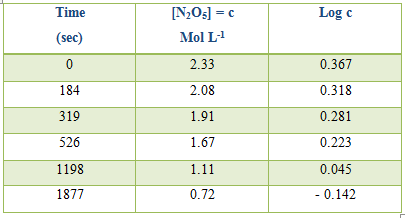Chemistry

# Typical Reactions: First-order ReactionTypical Reactions: First-order Reaction

First-order reaction is a chemical reaction in which the rate of reaction is fully proportional to the concentration of the reacting substance — compare order of a reaction. Example:

The thermal decomposition of nitrogen pentoxide in:

N2O5 → N2O4 + ½ O2

the gas phase and also in solution is an excellent example of a first order reaction. The reaction has been studied extensively and it has been found to obey first-order kinetics strictly over a wide range of concentration and temperature. The reaction also proceeds in CCl4 solution and the N2O4 remains in solution whereas oxygen is liberated and goes out as gas due to its very km solubility in carbon tetrachloride. The reaction, therefore, can be easily followed by measuring the volume of oxygen liberated at measured time intervals. In this case the raw expression becomes;

k = [2.303/(t2-t1)] log [(V -V1) – (V -V1)]

Where V is the volume of oxygen evolved after infinite time, V1 and V2 are the volumes of oxygen evolved at time t1 and t2 as V – V is proportional to concentration of N2O5 in CCl4.

Table: Decomposition of N2O5 in CCl4 at 300Ck (mean value) = 8.1 x 10-5 sec

Alternatively log c, where c is the concentration, can be plotted against time and k can be evaluated from the slope of the straight line.

The decomposition of the pentoxide in gas phase is somewhat complicated due to the equilibrium N2O4 (g) ↔ 2NO2 (g).

Table: Decomposition of N2O5 in CCl4 at 450Ck (mean value) = 6.2 x 10-2 sec.